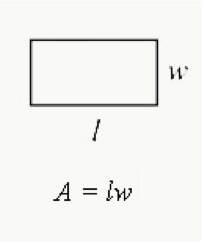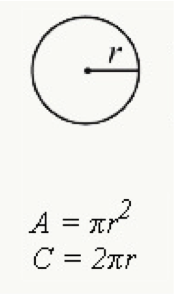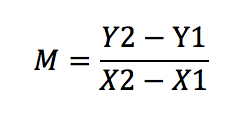# GRE Math Formulas

Most Common GRE Math Equations Cheat Sheet

##What GRE Formulas Should Students Know and Memorize?

The GRE Math section covers various topics, including coordinate systems, tables, symbols, and shapes.

Therefore, it is essential to know what is expected of you during the exam to help you get familiar with the vital formulas to acing the test.

Here is a review of the Math/Quantitative reasoning formulas on the GRE exam that you should memorize.

## GRE Math Section Topics

The math section of GRE is called Quantitative Reasoning or Quant, and it is among the three sections tested in GRE General Test. There are two Quant sections within each comprising 20 questions that you must complete within 35 minutes per section.

You will have an on-screen calculator if you are doing the computer-based test, and if you are doing the paper-delivered test, a calculator will be provided at the Prometric center. To many people, this is the toughest GRE section.

The quant section covers math concepts covered in middle school and high school, and there is no testing of upper-level concepts such as trigonometry. However, there are four main topics covered in Quant: algebra, arithmetic, data analysis, and geometry.

### Algebra

Algebra includes where unknown and known numbers come into play. Concepts tested include inequalities, equations, expressions, constants and variables, functions, coordinate geometry, coordinate systems, factorizations, and lines & slopes.

### Arithmetic

Arithmetic is the foundation of all that one learns in mathematics, and it is basically number manipulation. The quant section primarily deals with multiplication, division, addition, and subtraction. Topics covered include absolute numbers, square roots & exponents, prime numbers, order of operations, fractions, decimals & integers, sequences, percentage changes, and ratios & proportions.

### Geometry

Geometry deals mostly with angles and shapes. Questions cover types of angles, angle relationships, parallel & perpendicular lines, circles, and sectors & arcs. Other topics are polygons, including rectangles, squares, trapezoids, triangles & parallelograms, perimeter, area, circumference & surface area, and solids and similar shapes.

### Data analysis

Data analysis involves interpreting data, and questions will be on frequency & data distribution, standard deviation, factorials, combinations & permutations, Percentiles & quartiles, statistics, graphs, probability, and normal distribution.

## What Common GRE Quant Formulas Should I Memorize?

It is vital to memorize Math formulas if you want to get a good score in your GRE Quant section. Understanding the formulas and knowing when to apply them will help you answer questions quickly and accurately, leading to a high score.

In addition, a high score in Quant can enhance your chances of admission into graduate school. The equations are sometimes then key to solving some quant problem. Unfortunately, if you don’t remember a quant formula, it will be hard for you to answer the question, and you may end up picking the wrong answer.

Although, at times, memorizing the math formulas is not necessary to solve a problem, having a grasp of the equations can save time and complex calculations. You are pacing against time, and you shouldn’t spend a lot of time doing one question.

## List of GRE Math Formulas

Here is a list of 10 GRE math formulas you must know for the GRE test:

1. Area of a Triangle
2. Pythagorean Theorem
3. Area of a Rectangle
4. Area of a Circle
5. Area of a Trapezoid
6. Area of a Cylinder
7. Slope Formula
8. Integration Equations
9. Differentiation Formulas
10. Sin, tan, cot

## GRE Math Formula Cheat Sheet

Here is a cheat sheet for memorizing the math formulas on the GRE test.

Each section is broken down by topic appearing on the exam including geometry, algebra, calculus, & differentiation.

### GeometryTriangle

The area of a triangle is calculated by:

Area = ½ base x height (½ bh)

Where a is the base and b is the height

Pythagoras theorem is used to calculate the length of a side of a right-angled triangle. The square of the longest side is equal to the sum of the two short sides.

The measure of interior angles of an n-sided polygon: (n-2)x180/n.Rectangle

The area of a rectangle is calculated by multiplying the length and width.

A= length x width (lw) or A=½(diagonal)²

The perimeter of a rectangle is the sum of the length and width multiplied by 2

P= 2(Length + Width)Circle

The area of a circle is calculated by multiplying pi (π) with radius (r2)

Area = πr2

Where is π is a constant represented by 3.14159 and r is half the length of the line cutting the circler (diameter) into two halves

Circumference of a circle is the distance around the circle, calculated by multiplying pi with the diameter.

Circumference = πD or 2πrTrapezoid

Area of a Trapezoid: A=½(base₁+base₂)(height).

Area = ½ (a+b)h

This is a common math formula on the GRE test that is misunderstood. Pay close attention to how to use this on different types of problems.Cylinder

The surface area of a cylinder with top and bottom.

Area = 2πrh+2πr²

Where π= 3.14159, r is the radius of the cylinder and h is the height of the cylinder

This formula appears in several different various on many practice problems.

### Algebra

Slope

The slope is the gradient of alone on represented by m, which is a function of the change on the y-axis divided by change in the x-axis. If a line has two points (X1, Y1) and (X­2, Y2)Line equation is Y= MX =C

Where Y is they Coordinate and x is the x coordinate while M is slope and c is the Y-intercept

Distance between two points in a graph is √( x2- x1)+(y2-y1)

### Calculus

Integration is the process of continuous addition with a variable C representing the integration constant. The integral equations that you should recall to help you in Quant include:

xn dx = x(n+1) / (n+1) + C,(n -1)

bx dx = bx / ln(b) + C

sin x dx = -cos x + C

tan x dx = -ln|COs x| + C

### Differentiation

Differentiation involves finding a derivative of a function which is defined as Y=f(X). it is a measure of the rate of change of variable y relative to variable x change. Some of the differential formulas to remember include:

sin x = cos x

cot x = – csc2 x

cot h x = 1 – cot h2 x

sinh x = cosh x

## GRE Math & Quant Formula Study Tips

The main tip is knowing the basics by getting familiar with math concepts, rules, and formulas related to geometry, arithmetic, algebra, and data analysis.

Always try to memorize the standard formulas because they are vital to solving Quant problems. Unlike other standardized tests, there is no list of formulas for the GRE math section. Try to understand where the formulas apply to save time.

You can make flashcards to drill certain concepts and equations. Most importantly, you can use them in studying symbols and math terms. Equally, drilling realistic practice quant questions can help you master the formulas.

## Start Studying Today!

Don't put off preparing for the GRE Test. Start studying today and improve your score!